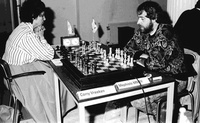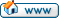ProDeo Would you like to react to this message? Create an account in a few clicks or log in to continue.ProDeoComputer ChessHomeCalendarLatest imagesFAQSearchMemberlistUsergroupsRegisterLog in

 ProDeo :: Computer Chess

# REBEL with Jeroen Noomen Opening Book3 posters
AuthorMessagePosts : 2412
Join date : 2020-11-17
Location : NetherlandsSubject: REBEL with Jeroen Noomen Opening BookSat Aug 20, 2022 8:47 am Jeroen Noomen the previous book editor of REBEL has returned to his first love. Jeroen is a walking encyclopedia of chess openings, look what he did with only 243 kilobytes (1/4 Mb).http://rebel13.nl/windows/rebel-15.html#15.1aPreview. Mclane, adminx, Dio, Eelco and Ipmanchess like this postPosts : 2412
Join date : 2020-11-17
Location : NetherlandsSubject: Re: REBEL with Jeroen Noomen Opening BookSat Aug 20, 2022 11:10 am Released.I asked Jeroen to check if the book functions as it should. Mclane likes this postMclanePosts : 2753
Join date : 2020-11-17
Age : 56
Location : United States of Europe, Germany, Ruhr areaSubject: Re: REBEL with Jeroen Noomen Opening BookSat Aug 20, 2022 6:56 pm I changed the paths. And i had to run it via this opening book program. I did not use the whole arena stuff.Only copied the rebel.bin out of the arena book directory.I set ponder = on and increased hash from 128 to 512. threads depending on the machine i run it.It works. Will soon find out where it is in the top 10….Posts : 2412
Join date : 2020-11-17
Location : NetherlandsSubject: Re: REBEL with Jeroen Noomen Opening BookSat Aug 20, 2022 8:05 pm Mclane wrote: I changed the paths. And i had to run it via this opening book program. I did not use the whole arena stuff.Only copied the rebel.bin out of the arena book directory.I set ponder = on and increased hash from 128 to 512. threads depending on the machine i run it.It works. Will soon find out where it is in the top 10…. If it works then it works, but it must run via polyglot.exe and polyglot.ini. Mclane likes this postMclanePosts : 2753
Join date : 2020-11-17
Age : 56
Location : United States of Europe, Germany, Ruhr areaSubject: Re: REBEL with Jeroen Noomen Opening BookSat Aug 20, 2022 8:46 pm Yes. Because it is a polyglot book. Admin likes this postgerhard

Posts : 22
Join date : 2022-08-18Subject: Convert Rebel.binSun Aug 21, 2022 11:52 am Mclane wrote: Yes. Because it is a polyglot book. OpenBook does a great job converting bin to pgn and apk (Arena Book)Open Book from Graham O'Neill- its for usage in cmd-box adminx and Dio like this postPosts : 2412
Join date : 2020-11-17
Location : NetherlandsSubject: Re: REBEL with Jeroen Noomen Opening BookMon Aug 22, 2022 8:02 am gerhard wrote: Mclane wrote: Yes. Because it is a polyglot book. OpenBook does a great job converting bin to pgn and apk (Arena Book)Open Book from Graham O'Neill- its for usage in cmd-box Works like a charm, thank you.MclanePosts : 2753
Join date : 2020-11-17
Age : 56
Location : United States of Europe, Germany, Ruhr areaSubject: Re: REBEL with Jeroen Noomen Opening BookMon Aug 22, 2022 10:59 am Code:     Motor                                Punkte  St Dr Dr Be Et Dr Dr Ru Lc Re De Re Ko Wa Ko Ch Bo Eq De De CS In Co Na De SO    S-B01: Stockfish_15_x64_avx2 [t4]          41,5/50 ·· == == 11 =1 11 =1 =1 == 0= == =1 11 =1 =1 11 11 11 11 11 11 11 11 11 11 11  925,7502: Dragon-2.5-64bit-avx2 [t4, mcts]    40,5/50 == ·· =0 0= 1= == 11 1= 1= =1 10 == 11 11 1= 11 11 11 11 11 11 11 11 11 11 11  882,0003: Dragon-3.1-64bit-avx2 [t4]          39,5/50 == =1 ·· == =1 1= == == 10 11 == == 1= 11 11 11 =1 =1 11 11 11 11 11 1= 11 11  881,5004: Berserk-9-x64-avx2-pext [t4]        37,0/50 00 1= == ·· 11 == =1 =1 == == =1 1= 11 10 10 =1 11 01 =1 11 11 =1 11 11 11 11  817,5005: Ethereal-13.75-pext-avx2 [t4]        36,5/50 =0 0= =0 00 ·· == == 0= =1 =1 == == 11 11 1= 11 11 11 11 11 11 11 11 11 11 11  739,2506: Dragon-2.5-64bit-avx2 [t4]          35,0/50 00 == 0= == == ·· == 0= == == =1 11 1= 11 == 01 11 01 11 11 11 11 11 11 11 11  721,5007: Dragon-3.1-64bit-avx2 [t4, mcts]    35,0/50 =0 00 == =0 == == ·· == == 1= =0 1= == 1= 11 11 11 =1 11 1= 11 11 11 11 11 11  711,2508: RubiChess20220813_64-avx2 [t4, nnue] 34,0/50 =0 0= == =0 1= 1= == ·· =1 1= == == 01 =1 =1 == 11 11 01 11 11 01 =1 11 11 11  740,2509: Lc0 0.28.2 radeon gpu dx12   34,0/50 == 0= 01 == =0 == == =0 ·· == == == =0 1= =1 11 =1 =1 11 11 11 11 11 11 11 11  689,7510: Rebel-15.1a-avx2 [t4, rebel.bin]    33,5/50 1= =0 00 == =0 == 0= 0= == ·· == == =1 =1 11 0= 1= 11 11 11 11 11 11 11 11 11  672,7511: DeepHiarcs15.0 [t4]                  32,0/50 == 01 == =0 == =0 =1 == == == ·· =1 01 == == =1 == 11 =1 == =1 11 11 11 11 1=  689,5012: Rebel-15.1-avx2 [t4]                32,0/50 =0 == == 0= == 00 0= == == == =0 ·· =1 11 =1 == 11 11 =1 == =1 11 11 11 11 11  649,7513: Komodo-14.1-64bit [t4, mcts]        28,5/50 00 00 0= 00 00 0= == 10 =1 =0 10 =0 ·· =1 =1 11 =1 1= 01 11 10 11 11 11 11 11  525,5014: Wasp550-windows-avx [t4]            27,0/50 =0 00 00 01 00 00 0= =0 0= =0 == 00 =0 ·· 01 =1 1= 11 11 11 11 11 11 11 11 11  453,0015: Komodo-14.1-64bit [t4]              25,5/50 =0 0= 00 01 0= == 00 =0 =0 00 == =0 =0 10 ·· 10 10 10 11 11 01 11 11 11 11 11  452,0016: Chiron-5-64bit [t4]                  22,0/50 00 00 00 =0 00 10 00 == 00 1= =0 == 00 =0 01 ·· == =0 1= 1= == 1= 11 11 11 11  369,7517: Booot6 [t4]                          21,5/50 00 00 =0 00 00 00 00 00 =0 0= == 00 =0 0= 01 == ·· 01 =1 =1 11 11 11 11 11 11  314,0018: Equinox 3.30-x64 [t4]                21,0/50 00 00 =0 10 00 10 =0 00 =0 00 00 00 0= 00 01 =1 10 ·· 10 01 1= 11 11 11 11 11  329,5019: Deep Rybka 4.1 x64 [t4]              16,0/50 00 00 00 =0 00 00 00 10 00 00 =0 =0 10 00 00 0= =0 01 ·· =1 =0 1= 01 11 11 11  229,2520: DeepShredder13UCIx64 [t4, buffer6]  15,5/50 00 00 00 00 00 00 0= 00 00 00 == == 00 00 00 0= =0 10 =0 ·· 1= 11 11 10 11 11  204,7521: CSTal3 5'                            15,0/50 00 00 00 00 00 00 00 00 00 00 =0 =0 01 00 10 == 00 0= =1 0= ·· 11 01 11 =1 11  195,2522: Inanis 1.1.1 win64 [t4]              8,5/50  00 00 00 =0 00 00 00 10 00 00 00 00 00 00 00 0= 00 00 0= 00 00 ·· =1 10 =1 11  98,5023: Colossus2022a [t4]                  8,0/50  00 00 00 00 00 00 00 =0 00 00 00 00 00 00 00 00 00 00 10 00 10 =0 ·· 10 11 11  69,2524: Nawito-22.07-winx64                  5,0/50  00 00 0= 00 00 00 00 00 00 00 00 00 00 00 00 00 00 00 00 01 00 01 01 ·· == =0  56,7525: Delfi                                4,0/50  00 00 00 00 00 00 00 00 00 00 00 00 00 00 00 00 00 00 00 00 =0 =0 00 == ·· 11  20,7526: SOS 5.1 for Arena                    2,0/50  00 00 00 00 00 00 00 00 00 00 0= 00 00 00 00 00 00 00 00 00 00 00 00 =1 00 ··  23,50650 Partien gespielt / Turnier beendetName des Turniers: Ryzen7 5700Ort/ Land: LAPTOP-JTQQP8M7, DeutschlandSpielstufe: Turnier 40/4Hardware: AMD Ryzen 7 5700U with Radeon Graphics  mit 4.096 MB Speicher Admin likes this postSubject: Re: REBEL with Jeroen Noomen Opening BookREBEL with Jeroen Noomen Opening BookPage 1 of 1

Permissions in this forum:You cannot reply to topics in this forum